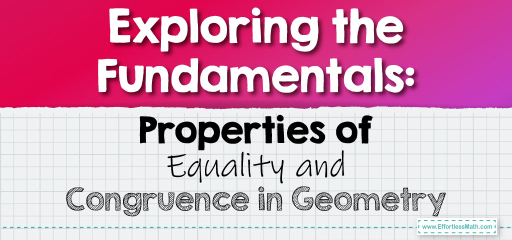# Exploring the Fundamentals: Properties of Equality and Congruence in Geometry

In math, we often talk about things being the same or equal, and that's where the properties of equality come in. They help us understand when and why numbers or expressions are equal. On the other hand, when we look at shapes and want to see if they're the same size and form, we talk about congruence. Both equality and congruence have certain rules or properties that help us work with them. Let's dive in and learn more about these important mathematical ideas.## Step-by-step Guide: Properties of Equality and Congruence

Properties of Equality
Equality properties form the basis of algebraic proofs and help justify the steps taken to solve equations. Here are the primary properties of equality:

• Reflexive Property: For any quantity $$a$$, $$a = a$$.
• Symmetric Property: If $$a = b$$, then $$b = a$$.
• Transitive Property: If $$a = b$$ and $$b = c$$, then $$a = c$$.
• Addition Property: If $$a = b$$, then $$a + c = b + c$$.
• Subtraction Property: If $$a = b$$, then $$a – c = b – c$$.
• Multiplication Property: If $$a = b$$, then $$ac = bc$$.
• Division Property: If $$a = b$$ and $$c ≠ 0$$, then $$\frac{a}{c} = \frac{b}{c}$$.

Properties of Congruence
In geometry, when two figures or parts of figures are of the same size and shape, they are said to be congruent. The properties of congruence validate the relationships between these figures:

• Reflexive Property: Any geometric figure is congruent to itself. For any segment $$AB$$, $$AB \cong AB$$.
• Symmetric Property: If segment $$AB \cong CD$$, then segment \ CD \cong AB \).
• Transitive Property: If $$AB \cong CD$$ and $$CD \cong EF$$, then $$AB \cong EF$$.

Applications in Geometry
Properties of equality and congruence are often used in conjunction to prove relationships in geometry, especially within geometric proofs. By establishing that certain angles or sides are congruent, and then applying properties of equality, we can deduce relationships between various parts of geometric figures.

### Examples

Example 1: Properties of Equality
Given the equations:
1) $$x + 7 = 15$$
2) $$y – 4 = 10$$
Find the values of $$x$$ and $$y$$.

Solution:
1) For the first equation, using the subtraction property of equality:
$$x + 7 – 7 = 15 – 7$$
$$x = 8$$
2) For the second equation, using the addition property of equality:
$$y – 4 + 4 = 10 + 4$$
$$y = 14$$
Answer: $$x = 8$$and $$y = 14$$

Example 2: Properties of Congruence
Consider two triangles, Triangle $$ABC$$ and Triangle $$DEF$$. If:
1) Side $$AB$$ is congruent to side $$DE$$.
2) Side $$BC$$ is congruent to side $$EF$$.
3) Angle $$A$$ is congruent to Angle $$D$$.
Is Triangle $$ABC$$ congruent to Triangle $$DEF$$ based on the given information?

Solution:
Using the Side-Angle-Side (SAS) postulate of congruence, if two sides and the included angle of one triangle are congruent to two sides and the included angle of another triangle, then the two triangles are congruent.
Given:
1) Side $$AB$$ is congruent to side $$DE$$.
2) Angle $$A$$ is congruent to Angle $$D$$.
3) Side $$BC$$ is congruent to side $$EF$$.
Based on the SAS postulate, Triangle ABC is congruent to Triangle $$DEF$$.
Yes, Triangle $$ABC$$ is congruent to Triangle $$DEF$$.

### Practice Questions:

1. If $$a = b$$ and $$b = 7$$, what is $$a$$ based on the properties of equality?
2. Given segment $$XY \cong ST$$ and segment $$ST \cong UV$$, what can you conclude about segments $$XY$$ and $$UV$$?
3. If two angles are each congruent to $$45^\circ$$, are the two angles congruent to each other?

1. $$a = 7$$ (By the Transitive Property of Equality)
2. Segment $$XY$$ is congruent to segment $$UV$$ (By the Transitive Property of Congruence).
3. Yes, the two angles are congruent to each other (By the Transitive Property of Equality).

### What people say about "Exploring the Fundamentals: Properties of Equality and Congruence in Geometry - Effortless Math: We Help Students Learn to LOVE Mathematics"?

No one replied yet.

X
30% OFF

Limited time only!

Save Over 30%

SAVE $5 It was$16.99 now it is \$11.99